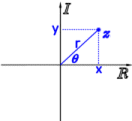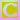Go to content
Since complex numbers have two parts - their real and imaginary parts - they can be plotted on a graph. The real part is plotted along the horizontal x-axis and the imaginary part is plotted on the vertical y-axis. Such a diagram is known as an Argand diagram.
This Argand diagram shows the modulus, |a| or |OA|, and the argument, \arg(a), shown in degrees, of the complex number a. If the coordinates of the point A are (b,c) then
a = b+ic
|a| = \sqrt{b^2+c^2} and
arg(a) = \tan^{-1}\displaystyle \frac{c}{b}

The modulus |OA| is the length of the line segment OA.
The argument arg(a) is measured anticlockwise from the positive real axis
Please enable Java for an interactive construction (with Cinderella).

## Summary/BackgroundAn Argand diagram is a plot of complex numbers, z=x+iy, as points in the complex plane using the x-axis as the real axis and y-axis as the imaginary axis.
While Jean-Robert Argand (1768 - 1822) is generally credited with the discovery in 1806, the Argand diagram (also known as the Argand plane) was actually described by C. Wessel prior to Argand. Historically, the geometric representation of a complex number as a point in the plane was important because it made the whole idea of a complex number more acceptable. In particular, this visualization helped "imaginary" and "complex" numbers become accepted in mainstream mathematics as a natural extension to negative numbers along the real line.Uses Cinderella interactive geometry

## Glossary

### argand diagram

A geometrical presentation of a complex number; using a real and imaginary axis to plot a+ib as (a,b).

### argument

On an argand diagram the angle between the complex number and the real axis.

### axis

One of two straight lines on a graph from which measurements are taken. One axis (the y axis) is vertical; the other (the x axis) is horizontal.

### complex number

A number of the form a+bi where i is the square root of -1, and a and b are real.

### geometric

A sequence where each term is obtained by multiplying the previous one by a constant.

### graph

A diagram showing a relationship between two variables.
The diagram shows a vertical y axis and a horizontal x axis.

### modulus

Absolute value, |x|.

### plot

To mark a point on a graph accurately by using its coordinates.

Full Glossary List

## This question appears in the following syllabi:

SyllabusModuleSectionTopicExam Year
AQA A-Level (UK - Pre-2017)FP2Complex NumbersArgand diagram-
AQA AS Further Maths 2017Pure MathsComplex Number BasicsArgand Diagrams-
AQA AS/A2 Further Maths 2017Pure MathsComplex Number BasicsArgand Diagrams-
CBSE XI (India)AlgebraComplex Numbers and Quadratic EquationsArgand diagrams-
CCEA A-Level (NI)FP1Complex NumbersArgand diagram-
CIE A-Level (UK)P3Complex NumbersArgand diagram-
Edexcel A-Level (UK - Pre-2017)FP1Complex NumbersArgand diagram-
Edexcel AS Further Maths 2017Core Pure MathsArgand DiagramsArgand Diagrams-
Edexcel AS/A2 Further Maths 2017Core Pure MathsArgand DiagramsArgand Diagrams-
I.B. Higher Level1Complex NumbersArgand diagram-
Methods (UK)M3Complex NumbersArgand diagram-
OCR A-Level (UK - Pre-2017)FP1Complex NumbersArgand diagram-
OCR AS Further Maths 2017Pure CoreArgand Diagrams and LociArgand Diagrams-
OCR MEI AS Further Maths 2017Core Pure AArgand Diagrams and LociArgand Diagrams-
OCR-MEI A-Level (UK - Pre-2017)FP1Complex NumbersArgand diagram-
Pre-U A-Level (UK)7Complex NumbersArgand diagram-